Jiang, P., Zhang, Y., Shan, Z., and Zheng, Q. (2013). "Optimizing the extraction yield of polyprenols from needles of Cunninghamia lanceolata (Lamb.) Hook using response surface methodology and its antioxidative activities," BioRes. 8(1), 545-556.

#### Abstract

An improved optimization method was used, combining a single-factor experiment and Response Surface Methodology to optimize the medium for the extraction yield of polyprenols from the needles of Cunninghamia lanceolata (Lamb.) Hook . A three-factor, three-level Box-Behnken design was used with extraction temperatures, extraction times, and liquid-solid ratio as independent variables to understand and optimize the extraction yield of polyprenols. A mathematical model with a high coefficient of determination was obtained and could be employed to optimize polyprenols extraction. From the optimized values of extraction temperature 71.4 °C, extraction time 5.96 h, and liquid-solid ratio 9.3:1, the extraction yield of polyprenols was 1.22 ± 0.04% (N = 3), which agreed closely with the predicted value (1.27%). Besides, polyprenols were demonstrated to have a strong antioxidative ability in vitro. Scavenging percentages of superoxide radical and DPPH by polyprenols were up to 75.6% and 56.9%, respectively.

Optimizing the Extraction Yield of Polyprenols from Needles of Cunninghamia lanceolata (Lamb.) Hook using Response Surface Methodology and its Antioxidative Activities

Ping Jiang,a,* Yang Zhang,a Zongxing Shan,b and Qinghe Zheng a

An improved optimization method was used, combining a single-factor experiment and Response Surface Methodology to optimize the medium for the extraction yield of polyprenols from the needles of Cunninghamia lanceolata (Lamb.) Hook. A three-factor, three-level Box-Behnken design was used with extraction temperatures, extraction times, and liquid-solid ratio as independent variables to understand and optimize the extraction yield of polyprenols. A mathematical model with a high coefficient of determination was obtained and could be employed to optimize polyprenols extraction. From the optimized values of extraction temperature 71.4 ℃, extraction time 5.96 h, and liquid-solid ratio 9.3:1, the extraction yield of polyprenols was 1.22 ± 0.04% (N = 3), which agreed closely with the predicted value (1.27%). Besides, polyprenols were demonstrated to have a strong antioxidative ability in vitro. Scavenging percentages of superoxide radical and DPPH by polyprenols were up to 75.6% and 56.9%, respectively.

Keywords: Cunninghamia lanceolata (Lamb.) Hook; Polyprenols; Single-factor experiment; Response surface methodology; Antioxidative ability

Contact information: a: Chemical processing engineering of forest products, Nanjing Forestry University, Nanjing, Jiangsu 210000, China; b: Biochemical Engineering, Nanjing Forestry University, Nanjing, Jiangsu 210000, China; * Corresponding author: cq_cheng@hotmail.com

INTRODUCTION

Cunninghamia lanceolata (Lamb.) Hook. is an important native tree species that has been widely planted in mountainous areas in the tropics and subtropics in China for more than 1,000 years and has been used for a variety of wood products. Also, in Chinese folklore medicine, the roots, branches, leaves, and bark of C. lanceolata have been used orally to cure arthralgia, stomach ache, and winter cough, and externally used for fractures, burns, scalds, and sensitization dermatitis (Xiu et al. 2012). Polyprenols (PP) are unsaturated acyclic branched alcohols having a primary hydroxyl group at the isoprene terminus. The quantity and geometric configuration of the units varies depending on the plant family. PP exists widely in green needles. They consist of a series of isoprene alkenyl and isoprene enolate units and possess a wide spectrum of biological activity. It was noted that needles of European fir contain PP (Khidyrora et al. 2002), so the abundant China fir resources were the main raw material in the present study. Polyprenols are metabolized in the human body into dolichols (Fig. 1). Exogenous polyprenols supplement the lack of dolichols naturally found in the body and play a significant role in immune function against multiple sclerosis and gout, as well as liver regeneration, suppression of cancer metastasis, and adjuvant radiotherapy (Wang et al. 2001). Thus, PP from plants are important intermediates of dolichols drugs. PP from pine needles have been approved for use in the production of healthy food and drugs in some European countries and the production of the drug, ROPREN, which is mainly used for immune dysfunction caused by a series of diseases. There are good prospects for application of polyprenols production in future.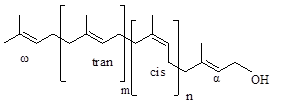Fig. 1. (a) Structure of polyprenols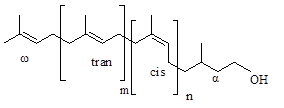Fig. 1. (b) Structure of dolichols

So far, plant polyprenols can be divided into the structure of ω-t3-cn-OH (n = 4 ~ 8) ficaprenol (Wellburn et al. 1967), ω-t2-cn-OH (n = 5) betulaprenol (Lindgren 1965), and all trans-solanesol ω-tm-OH (m = 7) (Rowland et al. 1956). We analyzed the polyprenols of the long-chain structure from more than 100 kinds of gymnosperms, of which about 60 species of coniferous plants polyprenols, in the form of ethyl acetate and the prenyl unit, number from 12 to 30. Most of them are of the betulaprenol type (Swiezewska and Chojnacki 1988). The content of polyprenols varies with different plants. Polyprenol and ester content of different pine and cypress needles varies between 0 to 1.2% (Ibata et al. 1983). Furthermore, the content of polyprenols from the same kind of plants changes with the season, humidity, location, and the environmental conditions (Wang et al. 2000).

Response Surface Methodology (RSM) has been applied as a powerful tool for optimal extraction of functional compounds from plant materials (Meng et al. 2009). The lack-of-fit test measures the failure of the model to represent the data in the experimental domain at points which are not included in the regression. The shapes of the contour plots, circular or elliptical, indicate whether the mutual interactions between the variables are significant or not. Circular contour plot indicates that the interactions between the corresponding variables are negligible, while elliptical contour plot indicates that the interactions between the corresponding variables are significant (Muralidhar et al. 2001). Types of RSM designs vary and include central composite design (CCD), Box-Behnken design (BBD), 3-level factorial design, and D-optimal design (Singh et al. 1995; Boza et al. 2000; Sanchez-Lafuente et al. 2002). The Box-Behnken design requires 17 runs based on a three-factor, three-level experimental design. In addition, the Box-Behnken design, a modified central composite experimental design, is an independent, rotatable or nearly rotatable quadratic design, in which the treatment combinations are at the midpoints of the edges of process space and at the center (Liu et al. 2012; Ragonese et al. 2002). Hence, the Box-Behnken design was applied to optimize the reaction parameters that play important roles in modification of the extraction yield of polyprenols. Based on the single-factor experiments, the design was used to estimate and optimize the extraction temperature, extraction time, and liquid-solid ratio. All the independent variables had highly significant effects on the response values. Thus, the main objective of this study was to maximize the yield of polyprenols by optimizing the extraction parameters by RSM. The study also investigated the interaction between different extraction parameters.

MATERIALS AND METHODS

Material

Needles of C. lanceolata (Lamb.) Hook were selected at Nanjing Forestry University. Dust was cleared from the needles, the needles were cut into pieces, and then the materials were air-dried by equilibration with ambient air at 25 ℃ and 50% relative humidity. All chemicals used in this experiment were of analytical grade and purchased from Nanjing Chemical Reagent Co, LTD. (Nanjing, China).

Polyprenols Extraction, Saponification, and Purification

The air-dried needles were extracted with ethyl acetate under a constant temperature provided by a water bath and then filtered. The filtered liquor was evaporated to dryness under reduced pressure. 5% NaOH of methanol solution (25 mL) and water (50 mL) were added as saponification solution, and the saponification reaction was accomplished at 40 oC for 4h. The free PP were produced by alkaline hydrolysis in aqueous alcoholic NaOH. Then, the solids were extracted with hexane (50 mL) twice for the fat-soluble layer. In this process, triglyceride, higher fatty acid, chlorophyll, etc., were removed after the reaction in the water-soluble phase. PP and some fat soluble impurities were in the fat-soluble layer. Anhydrous Na2SO4 (5 g) was added to the fat-soluble layer, the mixture was filtered, the solution was concentrated under reduced pressure, and then the crude product of PP was obtained.

Silica Gel Column Chromatography and HPLC

Silica gel column chromatography was used to further purify the sample for polyprenols; chromatography conditions were as follows: eluent, petroleum ether: hexane=7:1(by vol); flow velocity, one drop of eluent per second; silica gel specification, 100 to 200 mesh; silica gel column specification, 25×500 mm. The purification products were light yellow oily matter, and these samples were subjected to HPLC analysis.

HPLC of polyprenols was performed on a Agilent Zorbax SB-C18, 5 μm column, 4.6×150 mm, 215 nm using a Waters apparatus and a linear gradient from 30% to 80% of a mixture of hexane/isopropanol-6:4 in a mixture of hexane/methanol/isopropanol/water (9:27:54:10, by vol.). The wavelength of the UV–Vis detector was set at 215 nm.

Single Factor Experimental Design

The effect of extraction temperature, extraction time, and liquid-solid ratio on the yield of PP from C. lanceolata (Lamb.) Hook was first studied with a single-factor experimental design. One factor is changed, while the other factors were kept constant in each experiment. The effect of each factor was evaluated by determining the yield of PP.

BBD Experimental Design

Based on single-factor experiments, extraction temperature (X1), extraction time (X2), and liquid-solid ratio (X3) were selected for further optimization by RSM because of their significant effects on polyprenols extraction yield. There were a total of 17 runs for optimizing the three individual parameters in the selected Box–Behnken design (Table 1). Equation (1) represents the mathematical model relating to the independent parameters variables, Xi and the second-order polynomial coefficient for each term of the equation determined through multiple regression analysis using the Design Expert version 8.0.6. A second-order polynomial model was fitted to the experimental results for the yield of polyprenols according to the regression results of BBD:

Y = 1.24 + 0.054X– 0.22X1+ 0.097X– 0.20X2+ 0.059X– 0.11X3– 0.027X1X+ 0.050X1X3– 2.500E – 003X2X3 (1)

A BBD matrix of the independent variables in the form of a code along with responses of each experimental trial is given in Table 1.

Table. 1.　Box-Behnken Design and the Results of the Experiments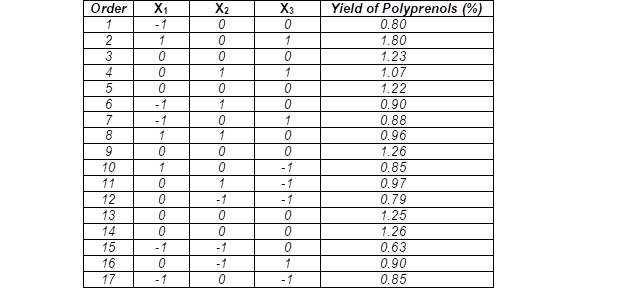Antioxidative Activity

DPPH radical scavenging experiments were carried out as described by Luo et al. (2009). Different concentrations of PP (0.2 to 1.2 mg/mL) were added to 2 mL DPPH solution 10.5 mol/L). The mixture was shaken and allowed to stand at 25 oC for 30 min in the dark. The absorbance value at 517 nm was measured against a blank (anhydrous ethanol replacing PP and DPPH solution, respectively). In Equation 2, A0 is an absorbance value of the control sample (anhydrous ethanol replacing PP of the mixture sample), A1 is an absorbance value of the mixture sample, and Ais an absorbance value of the control sample (anhydrous ethanol replacing DPPH solution of the mixture sample). The scavenging percentage was calculated as follows:

Scavenging percentage (%)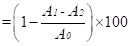(2)

Superoxide radical scavenging experiments were carried out as described by Yu and Chao (2012). The reaction mixture, including 4 mL Tris. HCl buffer (0.05 M, pH 8.2), pyrogallol (0.2 mM, 1 mL), and different concentrations of PP (0.2 to 1.2 mg/mL), was incubated at 25 oC for 20 min. After the incubation, the reaction mixture was detected by determining the absorbance value at 420 nm (A1). The PP sample was substituted with the distilled water as the control and the absorbance value at 420 nm was also detected (A2). The scavenging percentage of superoxide radical was calculated using the following equation:

Scavenging percentage (%)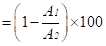(3)

Analytical Methods

Standard curve and regression equation

The polyprenols standard solution was scanned using the wavelength range from 200 to 700 nm of UV-visible light waves, and the maximum absorption was observed at 215 nm. Absorbance in the 215 nm wavelength, which is the absorption of polyprenols standard solution, increased as the concentration increased and showed a good linear relationship. As shown in Fig. 2, the concentration of polyprenols standard solution was approximately 0.04 to 0.16 mg·mL-1 within the scope, and the standard curve equation was as follows:

y = 3.51228x +1.96842 (R= 0.9954) (4)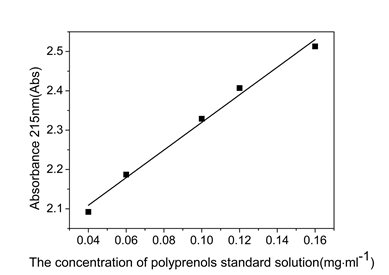Fig. 2. Specification curve of polyprenols’ absorption at 215 nm wavelength UV

Measuring the yield of polyprenols by UV-vis spectrophotometry

Assuming that the crude product of needles containing polyprenols is m, its diluents solution corresponding absorbance can be detected in the wavelength of 215 nm with a UV-visible spectrophotometer. According to the polyprenols standard equation y = a+ b, the concentration of crude product solution of polyprenols corresponding to the absorbance value y can be calculated, which works out the yield of polyprenols,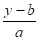= x ×Dilution factor

Y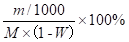(5)

where x is the concentration of the dilute solution of polyprenols crude product (with units of mg·mL-1), y is the absorbance of polyprenols crude solution diluted (abs.), m is the polyprenols quality of polyprenols crude solution (mg), M is the weight of the raw materials (g), is the moisture content of raw materials (%), and Y1 is the extraction yield of polyprenols (%).

RESULTS AND DISCUSSION

Single Factor Experimental Analysis

The results of single factor experiments are presented in Fig. 3. Three factors were found to have a significant impact on the yield of PP.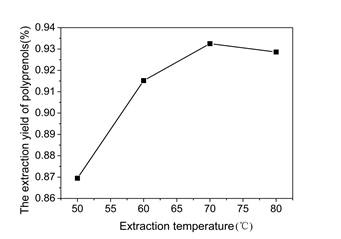A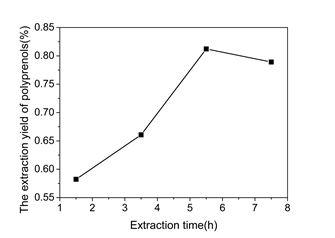B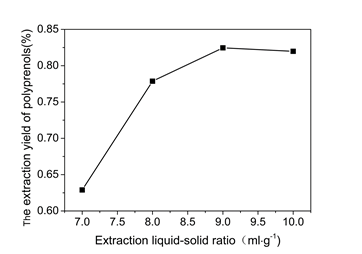C

Fig. 3. Effect of extraction temperature, extraction time and liquid-solid ratio on the yield of PP. (A) extraction temperature, (B) extraction time and (C) liquid-solid ratio. Extraction yield of PP are expressed as the mean of three measures.

To study the effect of different temperatures on the yield of PP, the extraction process was carried out at 50, 60, 70, and 80 oC. The yield of PP increased dramatically when extraction temperatures was increased from 50 to 70 oC. As shown in Fig. 3 (A), the maximal yield (0.93%) of PP was obtained at 70 oC. This tendency is in good agreement with some reports of other authors (Ray 2006; Vinogradov et al. 2003). The extraction time is another factor that affects the extraction efficiency and the selectivity of PP. The effect of different extraction times on the yield of PP is shown in Fig. 3 (B). A longer extraction time showed a positive effect on its yield. When the extraction time was varied from 1.5 to 5.5 h, the increase of the extraction yield was relatively rapid. The extraction yield of PP reached a maximal value of 0.82% at 5.5 h, and the yield of PP decreased slightly as the extraction time was prolonged beyond that point. This indicates that the extraction time of 5.5 h is sufficient to obtain a higher yield of PP. The effect of different liquid-solid ratio on the yield of PP is shown in Fig. 3 (C). The yield of PP increased significantly from 0.62% to 0.82 % when liquid-solid ratio was varied from 7:1 to 9:1 mg/mL. However, the yield of PP decreased when the ratio was over 9:1 mg/mL. A possible explanation is that with the increase of liquid-solid ratio, the PP that have been extracted have a synergistic effect on the rest of the PP present.

HPLC Analysis

As shown in Fig 4, the HPLC spectrum showed almost five familiar peaks between the standard products and the sample products within the retention time range from 1 to 4 minutes. It was noted that the sample isolated by silica gel column chromatography contain high purity PP. According to the area normalization method, the purity of PP from needles of C. lanceolata (Lamb.) Hook reached 96.3%.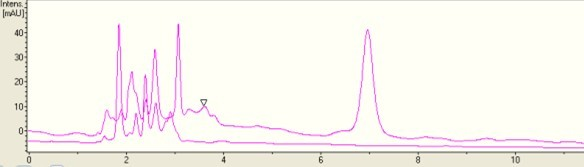Fig. 4. Polyprenols HPLC spectra of standard products (upper) and sample products (lower)

Optimization of Polyprenols Extraction Yield With Box-Behnken Design (BBD)

As shown in Table 2, the F-test suggested that the model had a very high F-value (F = 186.44) and a very low P-value (p < 0.001), indicating this model was highly significant. The F-value (1.24) and P-value (0.4063) of lack-of-fit implied that it was not significant relative to the pure error, which indicated that the model equation was adequate for predicting the yield of polyprenols under any combination of values of the variables. There is a 40.63% chance that a “Lack of fit F-value” this large could occur due to noise. R2 is a measure of the amount of variation around the mean explained by the model. It is the proportion of variation in the response attributed to the model rather than to random error. It has been suggested that for a well fitted model, Rshould not be less than 80%. The higher the R2 value, the higher the likelihood of fitting a model to the actual data (Koocheki et al. 2009). However, adding variables to the model will always increase R2, regardless of whether the additional terms are statistically significant or not. Thus, a large value of R2 cannot always imply the adequacy of the model. For this reason, it is more appropriate to use an adj-R2 of over 90% as a criterion of model adequacy. Adj-R2 is a measure of the amount of variation around the mean explained by the model, adjusted for the number of terms in the model. The adj-R2 decreases as the number of terms in the model increases, if those additional terms do not add substantial value to the model (Koocheki et al. 2009; Little and Hills et al. 1978). The value of the coefficient of determination (R2) calculated from the quadratic regression model was 0.9958, while the value of the adjusted determination coefficient (R2 Adj = 0.9905) was almost 1, indicating that non-significant terms have been included in the model and there was a high degree of correlation between the observed and predicted values.

Based on Fig. 5(a-f), the 3D response surface and 2D contour plots are the graphical representations of regression equation. They provide a method to visualize the relationship between responses and experimental levels of each variable and the type of interactions between two test variables. As shown in Fig. 5(a-f), the 3D response surface picture shows the opening downward concave curve, meaning the extraction yield has a maximum value. The yield of polyprenols was rapidly enhanced with the increasing of extraction temperature (X1), extraction time (X2) and liquid-solid ratio (X3) , while with the further increasing of extraction temperature (X1), extraction time (X2) and liquid-solid ratio (X3), the yield was slightly reduced. This suggested more yield resulted at higher extraction temperature, longer extraction time, and higher liquid-solid ratio within a certain range. Figure 5(d) shows that the shape of the response surface contour plot at various extraction temperatures (X1) and liquid-solid ratios (X3) is elliptical. It is indicated that interactions between the extraction temperature (X1) and liquid-solid ratio (X3) had significant effect.

Table 2. Variance Analysis for the Built Regression Model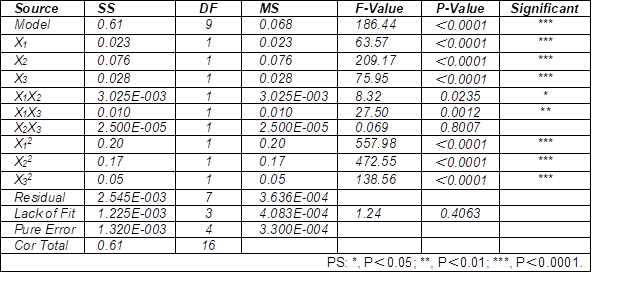The suitability of the model equation for predicting the optimum response values was tested using the selected optimal conditions. The predicted optimum conditions for polyprenols extraction were: extraction temperature of 71.4 oC, extraction time of 5.96 h, liquid-solid ratio of 9.3 mL·g-1, and the model predicted a maximum response of 1.27%. To ensure that the predicted results did not deviate from the practical value, experimental rechecking was performed using these modified optimal conditions: extraction temperature of 71 oC, extraction time of 6 h, and liquid-solid ratio of 9 mL·g-1. A mean value of 1.22 ± 0.04% (N = 3) was gained, which was in agreement with the predicted value and was obtained from real experiments. This demonstrated the validation of the RSM model. The results of analysis confirmed that the response model was adequate for reflecting the expected optimization, and the model of Eq. (1) was satisfactory and accurate.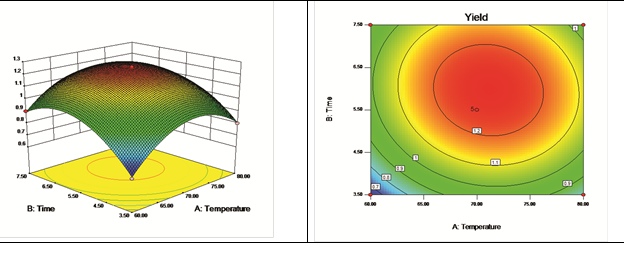a b

Fig. 5. (a) Response surface plots showing the effect of extraction temperature(X1) and extraction time (X2) on response yield; (b) Optimum conditions by contour plot of the responses evaluated as a function of extraction temperature (X1) vs. extraction time (X2)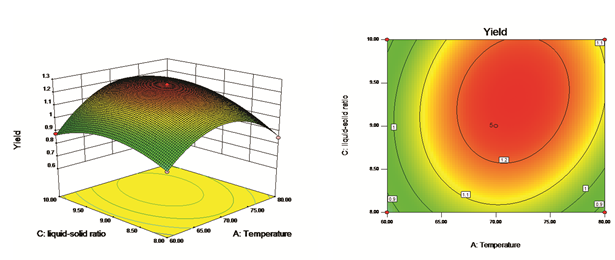c d

Fig. 5. (c) Response surface plots showing the effect of extraction temperature (X1) and liquid-solid ratio (X3) on response yield; (d) Optimum conditions by contour plot of the responses evaluated as a function of extraction temperature (X1) vs. liquid-solid ratio (X3)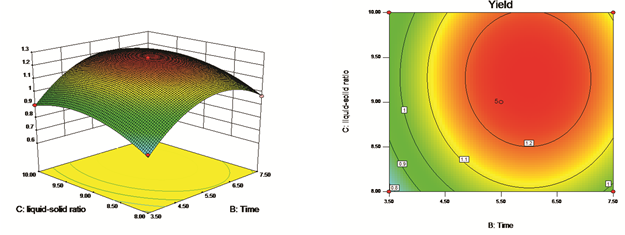e f

Fig. 5. (e) Response surface plots showing the effect of extraction time (X2) and liquid-solid ratio (X3) on response yield; (f) Optimum conditions by contour plot of the responses evaluated as a function of extraction time (X2) vs. liquid-solid ratio (X3)

In Vitro Antioxidative Ability of PP

As shown in Fig. 6, PP had a better antioxidative activity. The scavenging activity increased with an increase in the concentration of PP. At 1 mg/mL of PP, the scavenging activity of superoxide radical was 75.6%. The DPPH free radical is accepted widely as a tool for evaluating the free radical scavenging capacity of antioxidants. The scavenging activity of DPPH by PP is presented in Fig. 9. PP exhibited a strong scavenging ability to DPPH, and the scavenging percentage was 56.9% at 0.8 mg/mL. These results indicate that PP has a strong antioxidative ability and may be an alternative candidate for replacing synthetic antioxidants, such as BHA, BHT, and TBHQ.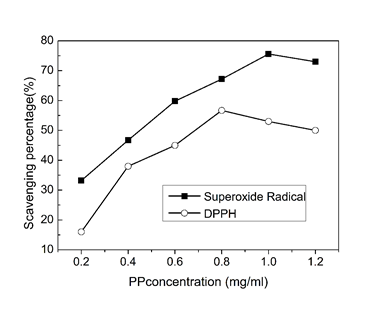Fig. 6. Scavenging percentages of free radicals by PP: superoxide radical and DPPH. Scavenging percentages are expressed as the mean of three measures

CONCLUSIONS

1. The extraction process of PP from C. lanceolata (Lamb.) Hook was optimized by Response Surface Methodology with a BBD design. A second-order polynomial model was established to depict the effect of three factors on the yield of PP. The optimal conditions for the extraction of PP were as follows: extraction temperature 71.4 oC, extraction time 5.96 h, and liquid-solid ratio 9.3 mg·mL-1. The maximal yield of PP was 1.22 ± 0.04% (N = 3) under the above conditions, which agreed closely with the predicted yield value.
2. A purity of PP as high as 96.3% was isolated by silica gel column chromatography, detected by HPLC. PP was demonstrated to have a strong antioxidative ability in vitro. These results indicate that PP may have a potential application as an effective component of pharmaceuticals in the future.

ACKNOWLEDGMENTS

This project was funded by the Priority Academic Program Development of Jiangsu Higher Education Institutions; Jiangsu Key Lab of Biomass-based Green Fuels and Chemicals.

REFERENCES CITED

Xiu, H. L., Zhai, X. F., Zheng, Xu., Wang, Y. L., and Wang, Z. (2012). “Anti-inflammatory and analgesic activity of total flavone of Cunninghamia lanceolata,” J. Molecules 17, 8842-8850.

Boza, A., De la Cruz, Y., Jordán, G., Jáuregui-Haza, U., Alemá, A., and Caraballo, I. (2000). “Statistical optimization of a sustained-release matrix tablet of lobenzarit disodium,” Drug Dev. Ind. Pharm. 26, 1303-1307.

Koocheki, A., Taherian, A. R., Razavi, S. M. A, and Bostan A. (2009). “Response surface methodology for optimization of extraction yield, viscosity, hue, and emulsion stability of mucilage extracted from Lepidium perfoliatum seeds,” Food Hydrocolloids 23, 2369-2379.

Lindgren, B. O. (1965). “Homologous aliphatic C30-C45 terpenols in birch wood,” J. Acta Chem. Scand . 19(6), 1319-1326.

Little, T. M., and Hills, F. J. (1978). Agricultural Experimentation Design and Analysis, John Wiley, NewYork.

Liu, J., Hu, H. R., Xu, J. F., and Wen, Y. B. (2012). “Optimizing enzymatic pretreatment of recycled fiber to improve its draining ability using response surface methodology,” BioResources. 7(2), 2121-2140.

Yu, P., and Chao, X. Y. (2012). “Statistics-based optimization of the extraction process of kelp polysaccharide and its activities,” Carbohydrate Polymers. 91, 356- 362

Meng, G. J., Lu, J., Fan, W., Dong, J. J., Zhang, J., Kong, W. B., Lin,Y., and Shan, L. J. (2009). “Optimization of extraction conditions for antioxidant phenolic compounds from malt rootlets using response surface methodology,” Journal of Food Biochemistry 33(2), 291-305.

Muralidhar, R. V., Chirumamilla, R. R., Ramachandran, V. N., Marchant, R., and Nigam, P. (2001). “Racemic resolution of RS-baclofen using lipase from Candida cylindracea,” Mededelingen. 66, 227-232.

Ragonese, R., Macka, M., Hughes, J., and Petocz, P. (2002). “The use of the Box-Behnken experimental design in the optimization and robustness testing of a capillary electrophoresis method for the analysis of ethambutol hydrochloride in a pharmaceutical formulation,” Journal of Pharmaceutical and Biomedical Analysis 27(6), 995-1007.

Luo, W., Zhao, M. M., Yang , B., Shen, G. L., and Rao, G. H. (2009). “Identification of bioactive compounds in Phyllenthus emblica L. fruit and their free radical scavenging activities,” Food Chemistry. 114(2), 499-504.

Ray, B. (2006). “Polysaccharides from Enteromorpha compressa: Isolation, purification and structural features,” Carbohydrate Polymers 66(3), 408-416.

Vinogradov, E. V., Brade, L., Brade, H., and Holst, O. (2003). “Structural and serological characterisation of the O-antigenic polysaccharide of the lipopolysaccharide from Acinetobacter baumannii strain 24,” J.Carbohydrate Research 338, 2751-2756.

Rowland, R., Latimer, P. H., and Giles, J. A. (1956). “Characterization of solanesol,” J. Amer. Chem. Soc. 78, 4680-4684.

Sánchez-Lafuente, C., Furlanetto, S., Fernández-Arévalo, M., Alvarez-Fuenyes, J., Rabasco, A. M., Faucci, M. T., Pinzauti, S., and Mura, P. (2002). “Didanosine extended-release matrix tablets: Optimization of formulation variables using statistical experimental design,” Int. J. Pharm. 237, 107-118.

Singh, S. K., Dodge, J., Durrani, M. J., and Khan, M. A. (1995). “Optimization and characterization of controlled release pellets coated with experimental latex: I. Anionic drug,” Int. J. Pharm. 125, 243-255.

Swiezewska, E., and Chojnacki, T. (1988) “Long-chain polyprenols in gymnosperm plants,” J. Acta Biochim. Polon. 35(2), 131-147.

Wang, C. Z., Shen, Z. B., Tan, W. H., and Yu, Q. (2000). “The content and seasonal variation of polyprenols from Ginkgo biloba L. leaves,” J. Plant Resources and Environment. 9(2), 57-58.

Wang, C. Z., Shen, Z. B., Tan, W. H., and Yu, Q. (2001). “The content analysis and application of polyprenols from Ginkgo biloba,” J. Chemistry and Industry of Forest Products. 35(5), 12-15.

Wellburn, A. R., Stevenson, J., Hemming, F. W., and Morton, R. A. (1967). “The characterization and properties of castaprenol-11, -12 and -13 from the leaves of Aesculus hippocastanum (horse chestnut),” J. Biochem. 102, 313-324.

Article submitted: September 14, 2012; Peer review completed: October 15, 2012; Revised version received and accepted: December 4, 2012; Published: December 7, 2012.